## RS Aggarwal Class 6 Solutions Chapter 4 Integers Ex 4E

These Solutions are part of RS Aggarwal Solutions Class 6. Here we have given RS Aggarwal Solutions Class 6 Chapter 4 Integers Ex 4E.

Other Exercises

Question 1.
Solution:
(i) 85 ÷ ( – 17) = $$\\ \frac { 85 }{ -17 }$$ = – 5
(ii) ( – 72) ÷ 18 = $$\\ \frac { -72 }{ 18 }$$ = – 4
(iii) ( – 80) ÷ 16 = $$\\ \frac { -80 }{ 16 }$$ = – 5
(iv) ( – 121) ÷ 11 = $$\\ \frac { -121 }{ 11 }$$ = – 11
(v) 108 ÷ ( – 12) = $$\\ \frac { 108 }{ -12 }$$ = – 9
(vi) ( – 161) ÷ 23 = $$\\ \frac { -161 }{ 23 }$$ = – 7
(vii) ( – 76) ÷ ( – 19) = $$\\ \frac { -76 }{ -19 }$$ = 4
(viii) ( – 147) + ( – 21) = $$\\ \frac { -147 }{ -21 }$$ = 7
(ix) ( – 639) ÷ ( – 71) = $$\\ \frac { -639 }{ -71 }$$ = 9
(x) ( – 15625) ÷ ( – 125) = $$\\ \frac { -15625 }{ -125 }$$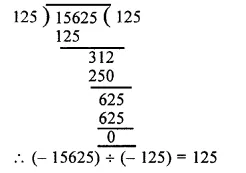(xi) 2067 ÷ ( – 1) = $$\\ \frac { 2067 }{ -1 }$$ = – 2067
(xii) 1765 ÷ ( – 1765) = $$\\ \frac { 1765 }{ -1765 }$$ = – 1
(xiii) 0 ÷ ( – 278) = $$\\ \frac { 0 }{ -278 }$$ = 0
(xiv) 3000 ÷ ( – 100) = $$\\ \frac { 3000 }{ -100 }$$ = – 30

Question 2.
Solution:
(i) 80 ÷ (…..) = – 5
Let 80 ÷ a = – 5
then, a = 80 ÷ ( – 5) = – 16
80 ÷ ( – 16) = – 5
(ii) – 84 + (…..) = – 7
Let – 84 ÷ a = – 7
then a = $$\\ \frac { -84 }{ -7 }$$ = 12s
– 84 ÷ 12 = – 7
(iii)(….) ÷ ( – 5) = 25
Let a + ( – 5) = 25
a = 25 x ( – 5) = – 125
( – 125) ÷ ( – 5) = 25
(iv)(……) ÷ 372 = 0
Let a ÷ 372 = 0
Then a = 6 x 372 = 0
(0) ÷ 372 = 0
(v)(….) ÷ 1 = – 186
Let a ÷ 1 = – 186
Then a = – 186 x 1 = – 186
( – 186) ÷ 1 = – 186
(vi)(…..) ÷ 17 = – 2
Let a ÷ 17 = – 2
Then a = – 2 x 17 = – 34
( – 34) ÷ 17 = – 2
(vii) (….) ÷ 165 = – 1
Let a ÷ 165 = – 1
Then a = – 1 x 165 = – 165
( – 165) ÷ 165 = – 1
(viii) (….) + ( – 1) = 73
Let a ÷ ( – 1) = 73
Then a = 73 ( – 1) = – 73
( – 73) + ( – 1) = 73
(ix) 1 ÷ (…..) = – 1
Let 1 ÷ (a) = – 1
Then a = – 1 x 1 = – 1
1 ÷ ( – 1) = – 1 Ans.

Question 3.
Solution:
(i) True : as if zero is divided by any non-zero integer, then quotient is always zero.
(ii) False : As division by zero is not admissible.
(iii) True : As dividing by one integer by another having opposite signs is negative.
(iv) False : As dividing one integer by another having the same signs is positive not negative.
(v) True : As dividing one integer by another with same sign is always positive.
(vi) True : As dividing one integer by another having opposite signs is always negative.
(vii) True : As dividing one integer by another having opposite signs is always negative.
(viii) True : As dividing one integer by another having opposite signs is always negative.
(ix) False : As dividing one integer by another having same signs is always positive not negative

Hope given RS Aggarwal Solutions Class 6 Chapter 4 Integers Ex 4E are helpful to complete your math homework.

If you have any doubts, please comment below. Learn Insta try to provide online math tutoring for you.

## RS Aggarwal Class 6 Solutions Chapter 21 Concept of Perimeter and Area Ex 21A

These Solutions are part of RS Aggarwal Solutions Class 6. Here we have given RS Aggarwal Solutions Class 6 Chapter 21 Concept of Perimeter and Area Ex 21A

Other Exercises

Question 1.
Solution:
(i) Length (l) = 16.8 cm
Perimeter = 2 (l + b)
= 2 (16.8 + 6.2) cm
= 2 x 23
= 46 cm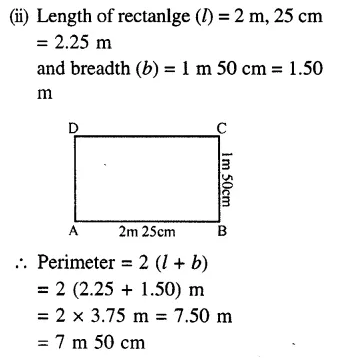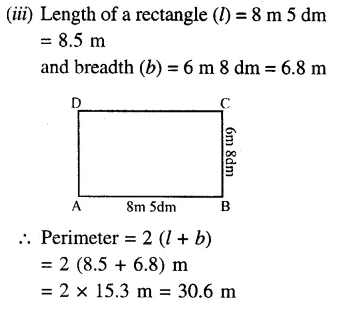= 30m 6 dm

Question 2.
Solution:
Length of rectangular field (l) = 62 m
and breadth (b) = 33 m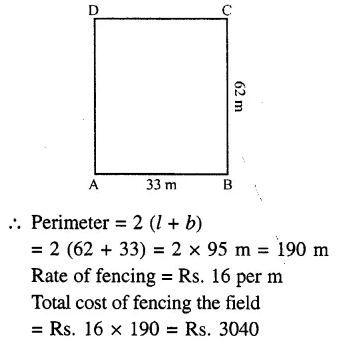Question 3.
Solution:
Perimeter of field = 128 m
Length + Breadth = $$\\ \frac { 128 }{ 2 }$$ = 64 m
Ratio in length and breadth = 5:3
Let length (l) = 5x
5x + 3x = 64
=> 5x = 64
=> x = $$\\ \frac { 64 }{ 8 }$$ = 8
Length of the field = 5x = 5 x 8 = 40m
and breadth = 3x = 3 x 8 = 24m

Question 4.
Solution:
Cost of fencing a rectangular field
= Rs. 18 per m
Total cost = Rs. 1980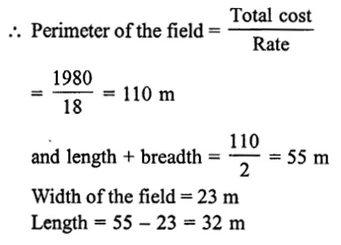Question 5.
Solution:
Total cost of fencing a rectangular field
= Rs 3300
Rate of fencing = Rs 25 per mQuestion 6.
Solution:
(i) Side of square = 3.8 cm
Perimeter = 4 x side
= 4 x 3.8 cm
= 15.2 cm
(ii) Side of a square = 4.6 m
Perimeter = 4 x side
= 4 x 4.6 m
= 18.4 m
(iii) Side of a square = 2 m 5 dm
= 2.5 m
Perimeter = 4 x side
= 4 x 2.5 m
= 10 m

Question 7.
Solution:
Total cost of fencing a square field = Rs. 4480
Rate of fencing = Rs. 35 per m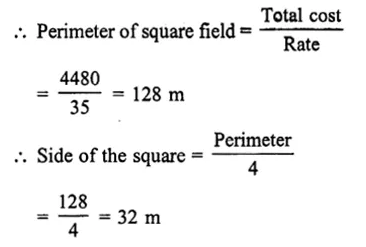Question 8.
Solution:
Side of a square field (a) = 21 m
Perimeter = 4a = 4 x 21 = 84m
Perimeter of rectangular field = 84 m
Ratio in length and breadth = 4 : 3
Let length (l) = 4x
Perimeter = 2 (l + b)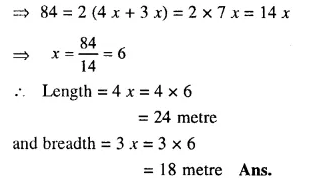Question 9.
Solution:
(i) Sides of a triangle are 7.8 cm, 6.5 cm and 5.9 cm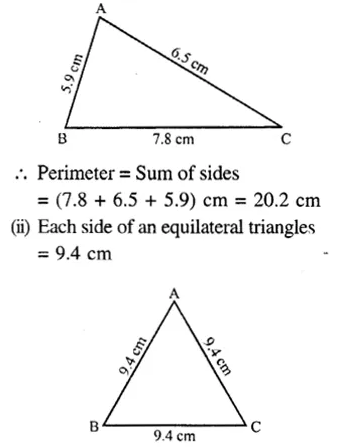Question 10.
Solution:
(i) Each side of a regular pentagon
= 8 cm
Perimeter = 5 x Side
= 5 x 8
= 40 cm
(ii) Each side of an octagon = 4.5 cm
Perimeter = 8 x Side
= 8 x 4.5
= 36 cm
(iii) Each side of a regular decagon = 3.6 cm
Perimeter = 10 x Side
= 10 x 3.6
= 36 cm

Question 11.
Solution:
We know that perimeter of a closed figure or a polygon = Sum of its sides
(i) In the figure, sides of a quadrilateral are 45 cm, 35 cm, 27 cm, 35 cm
Its perimeter = Sum of its sides
= (45 + 35 + 27 + 35) cm
= 142 cm
(ii) Sides of a quadrilateral (rhombus) are 18 cm, 18 cm, 18 cm, 18 cm
i.e., each side = 18 cm Perimeter
= 4 x Side
= 4 x 18
= 72 cm
(iii) Sides of the polygon given are 16 cm, 4 cm, 12 cm, 12 cm, 4 cm, 16 cm and 8 cm
Its perimeter = Sum of its sides
= (16 + 4 + 12 + 12 + 4 + 16 + 8) cm
= 72 cm

Hope given RS Aggarwal Solutions Class 6 Chapter 21 Concept of Perimeter and Area Ex 21A are helpful to complete your math homework.

If you have any doubts, please comment below. Learn Insta try to provide online math tutoring for you.

## RS Aggarwal Class 6 Solutions Chapter 20 Two-Dimensional Reflection Symmetry (Linear Symmetry) Ex 20

These Solutions are part of RS Aggarwal Solutions Class 6. Here we have given RS Aggarwal Solutions Class 6 Chapter 20 Two-Dimensional Reflection Symmetry (Linear Symmetry) Ex 20

Mark against the correct answer in each of Q. 1 to Q. 8.

Question 1.
Solution:
(d)∵ A square has four lines of symmetry, two diagonals and two lines joining the mid-points of opposite sides.

Question 2.
Solution:
(c) ∵ A rectangle has two lines of symmetry, each one of which being the line joining of mid-points of opposite sides.

Question 3.
Solution:
(b) ∵ A rhombus has two lines of symmetry namely two diagonals.

Question 4.
Solution:
(d) Each diameter of a circle is its line of symmetry which are unlimited numbers.

Question 5.
Solution:
(a) ∵ A scalene triangle has no line of symmetry.

Question 6.
Solution:
(a) ∵ It is a figure of kite ; so AC is its line of symmetry.

Question 7.
Solution:
(c) ∵ Letter O has two lines of symmetry, one vertical and second horizontal

Question 8.
Solution:
(a) ∵ Letter Z has no line of symmetry.

Question 9.
Solution:Question 10.
Solution:
(i) True (T) Parallelogram has no line of symmetry.
(ii) True (T) Bisector of an angle of*equal sides is the line of symmetry.
(iii) True (T)  Perpendiculars from each vertices’s of an equilateral-triangle to its opposite side is its line of symmetry.
(iv) False (F) Rhombus has two lines of symmetry which are its -diagonals.
(v) True (T) Square has four lines of symmetry, two diagonals and two perpendicular bisectors of opposite sides.
(vi) True (T) A rectangle has two lines of symmetry which are the perpendicular bisectors of its opposite sides.
(vii) True (T) H, I, O and X has two lines of symmetry.

Hope given RS Aggarwal Solutions Class 6 Chapter 20 Two-Dimensional Reflection Symmetry (Linear Symmetry) Ex 20 are helpful to complete your math homework.

If you have any doubts, please comment below. Learn Insta try to provide online math tutoring for you.

## RS Aggarwal Class 6 Solutions Chapter 19 Three-Dimensional Shapes Ex 19

These Solutions are part of RS Aggarwal Solutions Class 6. Here we have given RS Aggarwal Solutions Class 6 Chapter 19 Three-Dimensional Shapes Ex 19

Tick the correct answer in each of Q. 1 to Q. 6.

Question 1.
Solution:
(c) ∵ A cuboid has three dimensions, length, breadth and height or depth.

Question 2.
Solution:
(b) ∵ Its six faces arc of square.

Question 3.
Solution:
(d) ∵ Its shape is of a cylinder as it is round in shape on either sides/faces.

Question 4.
Solution:
(c) ∵ Football is round as sphere.

Question 5.
Solution:
(b) A brick has length, breadth and height.

Question 6.
Solution:
(d) ∵ Its shape is like a cone. Ans.

Question 7.
Solution:
(i) solid
(ii) 6, 12 and 18
(iii) opposite
(iv) sphere
(v) cube
(vi) 4, 8
(vii) 3, 6
(viii) 6, 3, 2, 9 Ans.

Question 8.
Solution:
(a) A cone:
(i) Conical cup
(ii) An ice cream cup
(iii) Conical tent house
(iv) Conical vessel.
(b) A cuboid :
(i) A book,
(ii) A brick,
(iii) a box,
(iv) a briefcase.
(c) A cylinder
(i) Circular pipe
(ii) A jar or tumbler
(iii) A round powder tin
(iv) Circular pillar.

Hope given RS Aggarwal Solutions Class 6 Chapter 19 Three-Dimensional Shapes Ex 19 are helpful to complete your math homework.

If you have any doubts, please comment below. Learn Insta try to provide online math tutoring for you.

## RS Aggarwal Class 6 Solutions Chapter 5 Fractions Ex 5F

These Solutions are part of RS Aggarwal Solutions Class 6. Here we have given RS Aggarwal Solutions Class 6 Chapter 5 Fractions Ex 5F.

Other Exercises

Find the difference:

Question 1.
Solution:
$$\frac { 5 }{ 8 } -\frac { 1 }{ 8 }$$
= $$\\ \frac { 5-1 }{ 8 }$$
= $$\frac { 4 }{ 8 }$$
= $$\frac { 4\div 4 }{ 8\div 4 }$$
= $$\frac { 1 }{ 2 }$$

Question 2.
Solution:
$$\frac { 7 }{ 12 } -\frac { 5 }{ 12 }$$Question 3.
Solution:
$$4\frac { 3 }{ 7 } -2\frac { 4 }{ 7 }$$
= $$\frac { 31 }{ 7 } -\frac { 18 }{ 7 }$$Question 4.
Solution:
$$\frac { 5 }{ 6 } -\frac { 4 }{ 9 }$$Question 5.
Solution:
$$\frac { 1 }{ 2 } -\frac { 3 }{ 8 }$$Question 6.
Solution:
$$\frac { 5 }{ 8 } -\frac { 7 }{ 12 }$$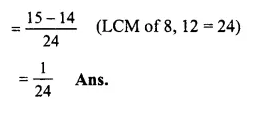Question 7.
Solution:
$$2\frac { 7 }{ 9 } -1\frac { 8 }{ 15 }$$
= $$\frac { 25 }{ 9 } -\frac { 23 }{ 15 }$$
(changing into improper fractions)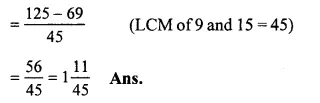Question 8.
Solution:
$$3\frac { 5 }{ 8 } -2\frac { 5 }{ 12 }$$
= $$\frac { 29 }{ 8 } -\frac { 29 }{ 12 }$$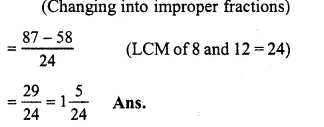Question 9.
Solution:
$$2\frac { 3 }{ 10 } -1\frac { 7 }{ 15 }$$
= $$\frac { 23 }{ 10 } -\frac { 22 }{ 15 }$$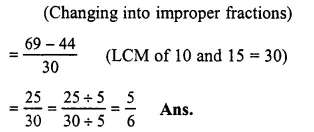Question 10.
Solution:
$$6\frac { 2 }{ 3 } -3\frac { 3 }{ 4 }$$
= $$\frac { 20 }{ 3 } -\frac { 15 }{ 4 }$$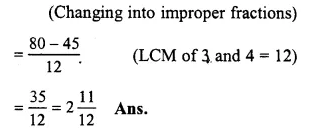Question 11.
Solution:
$$7-5\frac { 2 }{ 3 }$$
= $$\frac { 7 }{ 1 } -\frac { 17 }{ 3 }$$
(changing into improper fractions)Question 12.
Solution:
$$10-6\frac { 3 }{ 8 }$$
= $$\frac { 10 }{ 1 } -\frac { 51 }{ 8 }$$
(changing into improper fractions)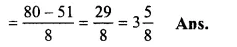Simpilify

Question 13.
Solution:
$$\frac { 5 }{ 6 } -\frac { 4 }{ 9 } +\frac { 2 }{ 3 }$$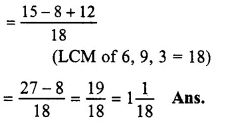Question 14.
Solution:
$$\frac { 5 }{ 8 } +\frac { 3 }{ 4 } -\frac { 7 }{ 12 }$$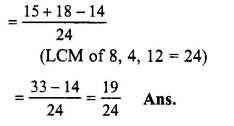Question 15.
Solution:
$$2+\frac { 11 }{ 15 } -\frac { 5 }{ 9 }$$
= $$\frac { 90+33-25 }{ 45 }$$
(LCM of 15 and 9 = 45)Question 16.
Solution:
$$5\frac { 3 }{ 4 } -4\frac { 5 }{ 12 } +3\frac { 1 }{ 6 }$$
= $$\frac { 23 }{ 4 } -\frac { 53 }{ 12 } +\frac { 19 }{ 6 }$$
(changing into improper fractions)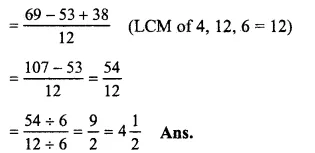Question 17.
Solution:
$$2+5\frac { 7 }{ 10 } -3\frac { 14 }{ 15 }$$
= $$\frac { 2 }{ 1 } +\frac { 57 }{ 10 } -\frac { 59 }{ 15 }$$
(changing into improper fractions)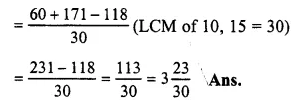Question 18.
Solution:
$$8-3\frac { 1 }{ 2 } -2\frac { 1 }{ 4 }$$
= $$\frac { 8 }{ 1 } -\frac { 7 }{ 2 } -\frac { 9 }{ 4 }$$
(changing into improper fractions)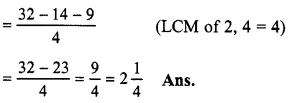Question 19.
Solution:
$$8\frac { 5 }{ 6 } -3\frac { 3 }{ 8 } +2\frac { 7 }{ 12 }$$
= $$\frac { 53 }{ 6 } -\frac { 27 }{ 8 } +\frac { 31 }{ 12 }$$
(changing into improper fractions)Question 20.
Solution:
$$6\frac { 1 }{ 6 } -5\frac { 1 }{ 5 } +3\frac { 1 }{ 3 }$$
= $$\frac { 37 }{ 6 } -\frac { 26 }{ 5 } +\frac { 10 }{ 3 }$$
(changing into improper fractions)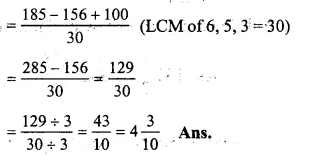Question 21.
Solution:
$$3+1\frac { 1 }{ 5 } +\frac { 2 }{ 3 } -\frac { 7 }{ 15 }$$
= $$\frac { 3 }{ 1 } +\frac { 6 }{ 5 } +\frac { 2 }{ 3 } -\frac { 7 }{ 15 }$$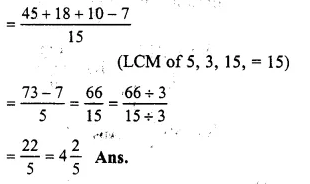Question 22.
Solution:
By subtracting $$9 \frac { 2 }{ 3 }$$ from 19, we get the required numberQuestion 23.
Solution:
By subtracting $$6 \frac { 7 }{ 15 }$$ from $$8 \frac { 1 }{ 5 }$$ we get the required numberQuestion 24.
Solution:
Sum of $$3 \frac { 5 }{ 9 }$$ and $$3 \frac { 1 }{ 3 }$$
= $$\frac { 32 }{ 9 } +\frac { 10 }{ 3 }$$Question 25.
Solution:
$$\\ \frac { 3 }{ 4 }$$, $$\\ \frac { 5 }{ 7 }$$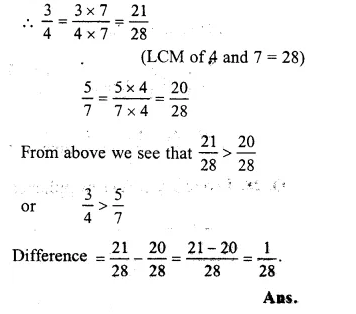Question 26.
Solution:
Milk bought by Mrs. Soni = $$7 \frac { 1 }{ 2 }$$ litres
and milk consumed by here = $$5 \frac { 3 }{ 4 }$$ litres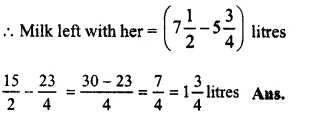Question 27.
Solution:
Total time of film show = $$3 \frac { 1 }{ 3 }$$ hours
Total spent on advertisement = $$1 \frac { 3 }{ 4 }$$ hours
Duration of the film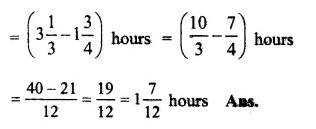Question 28.
Solution:
On a day, rickshaw pullar earned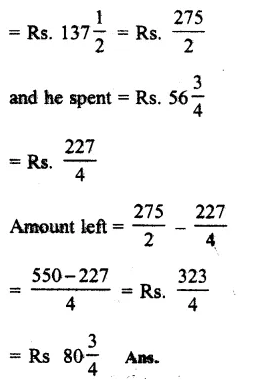Question 29.
Solution:
Total length of wire =$$2 \frac { 3 }{ 4 }$$-metres
Length of one piece = $$\\ \frac { 5 }{ 8 }$$ metre
Length of the other piece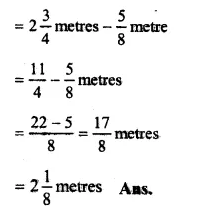Hope given RS Aggarwal Solutions Class 6 Chapter 5 Fractions Ex 5F are helpful to complete your math homework.

If you have any doubts, please comment below. Learn Insta try to provide online math tutoring for you.

## RS Aggarwal Class 6 Solutions Chapter 18 Circles Ex 18

These Solutions are part of RS Aggarwal Solutions Class 6. Here we have given RS Aggarwal Solutions Class 6 Chapter 18 Circles Ex 18

Question 1.
Solution:
Method :Take a point O on the paper as shown in the figure. With the help of the rular, open out compasses in such a way that the distance between the metal point and pencil point is 4 cm. Take the compasses in the same position and put its metal point at O and draw the circle.
Remove the compasses and again open out the compasses in such a way that the distance between the metal point and pencil point is 5.3 cm. Taking O as the centre, draw another circle. Again remove the compasses and similarly draw the third circle with radius 6.2 cm. Then the required circles are as shown in the figure which have radius OA = 4 cm., OB = 5.3 cm. and OC = 6.2 cm.

Question 2.
Solution:
Method : Take a point C on the paper. With the help of the rular, open out the compasses in such a way that the distance between its metal point and pencil point is 4.5 cm. Take the compasses in the same position and put its metal point at C and draw the circle. Mark points P, Q and R as shown in the figure as required.Question 3.
Solution:
Method : Take a point O on the paper. With the help of the rular, open out the compasses in such a way that the distance between the metal point and pencil point is 4 cm. Take the compasses in the same position and put the metal point at O and draw the circle.Take A and B any points on the circle and join AB. ThenAB is the chord of the circle. Mark points X and Y on the circle as shown. Then arc AXB and arc AYB are the required minor and major arcs respectively.

Question 4.
Solution:
(i) False
(ii) True
(iii) False
(iv) False
(v) True.

Question 5.
Solution:
Steps of construction :
(i) With centre O and radius 3.7 cm, draw a circle.
(ii) Take a point A on the circumference of the circle.
(iii) Join OA.
(iv) At O, draw another radius OB such that ∠AOB = 72° with the help of protractor. Then sector AOB is the required one.Question 6.
Solution:
(i) > (ii) < (iii) > (iv) >. Ans.

Question 7.
Solution:
(i) Passes through
(ii) at the centre, on the circle
(iii) chord
(iv) arc
(v) sector.

Hope given RS Aggarwal Solutions Class 6 Chapter 18 Circles Ex 18 are helpful to complete your math homework.

If you have any doubts, please comment below. Learn Insta try to provide online math tutoring for you.

## RS Aggarwal Class 6 Solutions Chapter 17 Quadrilaterals Ex 17B

These Solutions are part of RS Aggarwal Solutions Class 6. Here we have given RS Aggarwal Solutions Class 6 Chapter 17 Quadrilaterals Ex 17B

Other Exercises

Objective questions
Mark against the correct answer in each of the following :

Question 1.
Solution:
(c) ∵ Sum of angles of a quadrilateral is 360°.

Question 2.
Solution:
Sum of 4 angles of a quadrilateral = 360° and three angles of a quadrilateral are 80°, 70° and 120°
∵ Fourth angle = 360° – (80° + 70° + 120°)
= 360° – 270° – 90° (c)

Question 3.
Solution:
Sum of angles of a quadrilateral = 360°
The ratio in there four angles is 3 : 4 : 5 : 6Question 4.
Solution:
(d) Y Quadrilateral having one pair of parallel sides is a trapezium.

Question 5.
Solution:
(d) ∵ Quadrilateral having opposite sides parallel is called a parallelogram.

Question 6.
Solution:
(b) ∵ A trapezium having nonparallel sides equal is called an isosceles trapezium.

Question 7.
Solution:
(b) ∵ Diagonals of a rhombus bisect each other at right angles.

Question 8.
Solution:
(b) ∵ A square has four equal sides and also diagonals are equal.

Question 9.
Solution:
A quadrilateral having two pairs of equal adjacent sides but unequal opposite angles is called a kite. (c)

Question 10.
Solution:
A regular quadrilateral is a quadrilateral having equal sides and equal angles which is a square. (c)

Hope given RS Aggarwal Solutions Class 6 Chapter 17 Quadrilaterals Ex 17B are helpful to complete your math homework.

If you have any doubts, please comment below. Learn Insta try to provide online math tutoring for you.

## RS Aggarwal Class 6 Solutions Chapter 5 Fractions Ex 5E

These Solutions are part of RS Aggarwal Solutions Class 6. Here we have given RS Aggarwal Solutions Class 6 Chapter 5 Fractions Ex 5E.

Other Exercises

Find the sum :

Question 1.
Solution:
$$\frac { 5 }{ 8 } +\frac { 1 }{ 8 }$$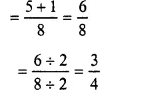Question 2.
Solution:
$$\frac { 4 }{ 9 } +\frac { 8 }{ 9 }$$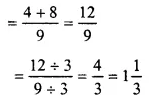Question 3.
Solution:
$$1\frac { 3 }{ 5 } +2\frac { 4 }{ 5 }$$
$$\frac { 8 }{ 5 } +\frac { 14 }{ 5 }$$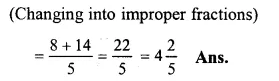Question 4.
Solution:
$$\frac { 2 }{ 5 } +\frac { 5 }{ 6 }$$
= $$\\ \frac { 4+15 }{ 18 }$$ (LCM of 9 and 6 = 18)
= $$\\ \frac { 19 }{ 18 }$$
= $$1 \frac { 1 }{ 18 }$$

Question 5.
Solution:
$$\frac { 7 }{ 12 } +\frac { 9 }{ 16 }$$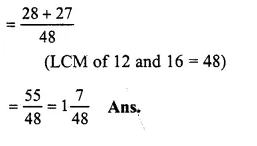Question 6.
Solution:
$$\frac { 4 }{ 15 } +\frac { 17 }{ 20 }$$Question 7.
Solution:
$$2\frac { 3 }{ 4 } +5\frac { 5 }{ 6 }$$
= $$\frac { 11 }{ 4 } +\frac { 35 }{ 6 }$$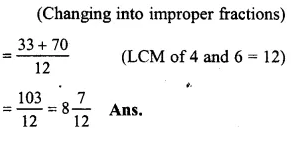Question 8.
Solution:
$$3\frac { 1 }{ 8 } +1\frac { 5 }{ 12 }$$
= $$\frac { 25 }{ 8 } +\frac { 17 }{ 12 }$$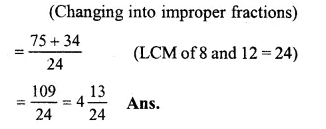Question 9.
Solution:
$$2\frac { 7 }{ 10 } +3\frac { 8 }{ 15 }$$
= $$\frac { 27 }{ 10 } +\frac { 53 }{ 15 }$$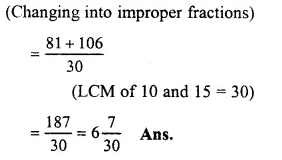Question 10.
Solution:
$$3\frac { 2 }{ 3 } +1\frac { 5 }{ 6 } +2$$
$$\frac { 11 }{ 3 } +\frac { 11 }{ 6 } +\frac { 2 }{ 1 }$$Question 11.
Solution:
$$3+1\frac { 4 }{ 15 } +1\frac { 3 }{ 20 }$$
=$$\frac { 3 }{ 1 } +\frac { 19 }{ 15 } +\frac { 23 }{ 20 }$$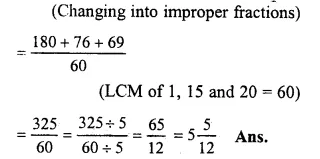Question 12.
Solution:
$$3\frac { 1 }{ 3 } +4\frac { 1 }{ 4 } +6\frac { 1 }{ 6 }$$
$$\frac { 10 }{ 3 } +\frac { 17 }{ 4 } +\frac { 37 }{ 6 }$$
(changing into improper fractions)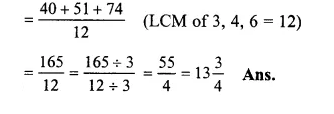Question 13.
Solution:
$$\frac { 2 }{ 3 } +3\frac { 1 }{ 6 } +4\frac { 2 }{ 9 } +2\frac { 5 }{ 18 }$$
$$\frac { 2 }{ 3 } +\frac { 19 }{ 6 } +\frac { 38 }{ 9 } +\frac { 41 }{ 18 }$$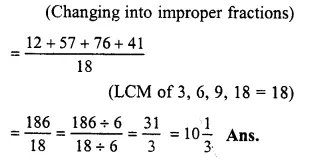Question 14.
Solution:
$$2\frac { 1 }{ 3 } +1\frac { 1 }{ 4 } +2\frac { 5 }{ 6 } +3\frac { 7 }{ 12 }$$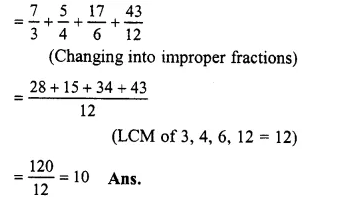Question 15.
Solution:
$$2+\frac { 3 }{ 4 } +1\frac { 5 }{ 6 } +3\frac { 7 }{ 16 }$$
$$\frac { 2 }{ 1 } +\frac { 3 }{ 4 } +\frac { 13 }{ 8 } +\frac { 55 }{ 16 }$$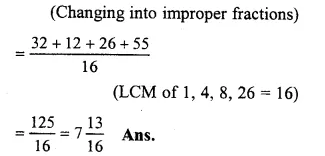Question 16.
Solution:
Cost of a pencil = Rs. $$3 \frac { 2 }{ 5 }$$
Cost of an eraser = Rs.$$2 \frac { 7 }{ 10 }$$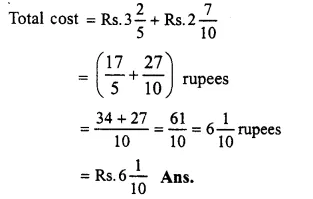Question 17.
Solution:
Length of cloth for kurta = $$4 \frac { 1 }{ 2 }$$ metres
Length of cloth for pyjamas = $$2 \frac { 2 }{ 3 }$$ metres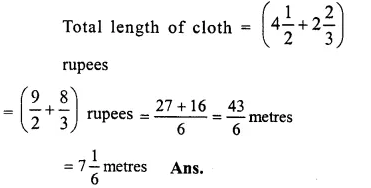Question 18.
Solution:
Distance travelled by Rickshaw = $$4 \frac { 3 }{ 4 }$$ km
Distance travelled on foot = $$1 \frac { 1 }{ 2 }$$ km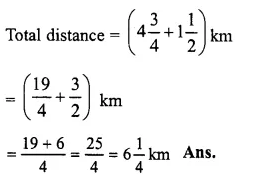Question 19.
Solution:
Weight of empty cylinder = $$16 \frac { 4 }{ 5 }$$ kg
Weight of gas filled in it = $$14 \frac { 2 }{ 3 }$$ kg
Total. weight of cylinder with gas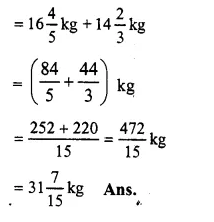Hope given RS Aggarwal Solutions Class 6 Chapter 5 Fractions Ex 5E are helpful to complete your math homework.

If you have any doubts, please comment below. Learn Insta try to provide online math tutoring for you.

## RS Aggarwal Class 6 Solutions Chapter 5 Fractions Ex 5D

These Solutions are part of RS Aggarwal Solutions Class 6. Here we have given RS Aggarwal Solutions Class 6 Chapter 5 Fractions Ex 5D.

Other Exercises

Question 1.
Solution:
(i) Like fraction : Fractions having the same denominators are called like fractions. For examples:
$$\frac { 2 }{ 11 } ,\frac { 3 }{ 11 } ,\frac { 4 }{ 11 } ,\frac { 5 }{ 11 } ,\frac { 8 }{ 11 }$$
(ii) Unlike fraction : Fraction having the different denominators, are called unlike fractions. For examples:
$$\frac { 1 }{ 3 } ,\frac { 4 }{ 7 } ,\frac { 5 }{ 9 } ,\frac { 3 }{ 8 } ,\frac { 7 }{ 11 }$$

Question 2.
Solution:
We know that like fractions have same denominator
Now $$\frac { 3 }{ 5 } ,\frac { 7 }{ 10 } ,\frac { 8 }{ 15 } ,\frac { 11 }{ 30 }$$
LCM of 5, 10, 15 and 30 = 30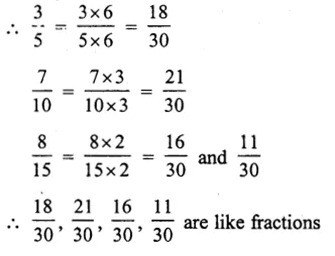Question 3.
Solution:
We know that like fraction have same denominators
$$\frac { 1 }{ 4 } ,\frac { 5 }{ 8 } ,\frac { 7 }{ 12 } ,\frac { 13 }{ 24 }$$
LCM of 4, 8, 12, 24 = 24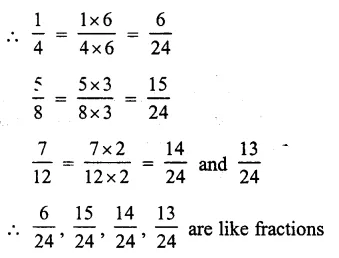Question 4.
Solution: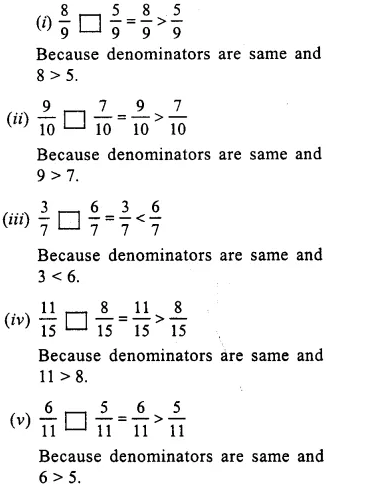Question 5.
Solution: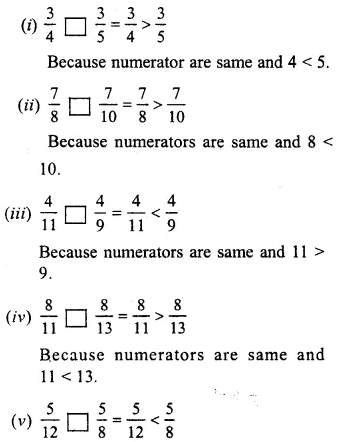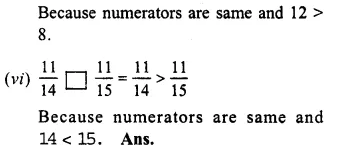Compare the fractions given below :

Question 6.
Solution:
$$\frac { 4 }{ 5 } and\frac { 5 }{ 7 }$$
LCM of 5 and 7 = 35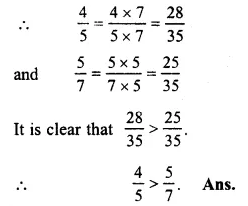Question 7.
Solution:
$$\frac { 3 }{ 8 } and\frac { 5 }{ 6 }$$
LCM of 8 and 6 = 24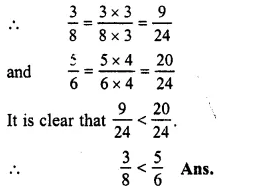Question 8.
Solution:
$$\frac { 7 }{ 11 } and\frac { 6 }{ 7 }$$
LCM of 11 and 7 = 77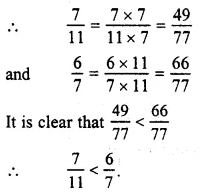Question 9.
Solution:
$$\frac { 5 }{ 6 } and\frac { 9 }{ 11 }$$
LCM of 6 and 11 = 66Question 10.
Solution:
$$\frac { 2 }{ 3 } and\frac { 4 }{ 9 }$$
LCM of 3 and 9 = 9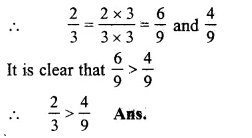Question 11.
Solution:
$$\frac { 6 }{ 13 } and\frac { 3 }{ 4 }$$
LCM of 13 and 4 = 52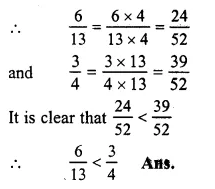Question 12.
Solution:
$$\frac { 3 }{ 4 } and\frac { 5 }{ 6 }$$
LCM of 4 and 6 = 12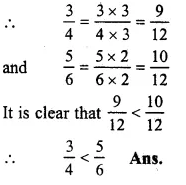Question 13.
Solution:
$$\frac { 5 }{ 8 } and\frac { 7 }{ 12 }$$
LCM of 8 and 12 = 24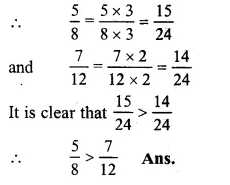Question 14.
Solution:
$$\frac { 4 }{ 9 } and\frac { 5 }{ 6 }$$
LCM of 9 and 6 = 18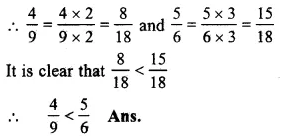Question 15.
Solution:
$$\frac { 4 }{ 5 } and\frac { 7 }{ 10 }$$
LCM of 5 and 10 = 10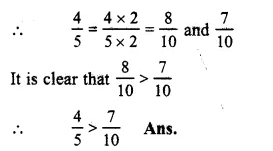Question 16.
Solution:
$$\frac { 7 }{ 8 } and\frac { 9 }{ 10 }$$
LCM of 8 and 10 = 40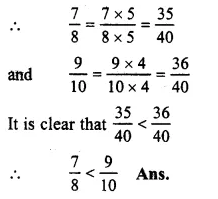Question 17.
Solution:
$$\frac { 11 }{ 12 } and\frac { 13 }{ 15 }$$
LCM of 12 and 15 = 60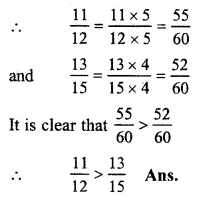Question 18.
Solution:
$$\frac { 1 }{ 2 } ,\frac { 3 }{ 4 } ,\frac { 5 }{ 6 } and\frac { 7 }{ 8 }$$
LCM of 2, 4, 6 and 8 = 24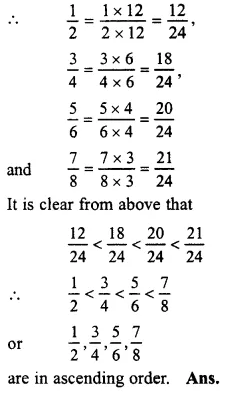Question 19.
Solution:
$$\frac { 2 }{ 3 } ,\frac { 5 }{ 6 } ,\frac { 7 }{ 9 } and\frac { 11 }{ 18 }$$
LCM of 3, 6, 9 and 18 = 18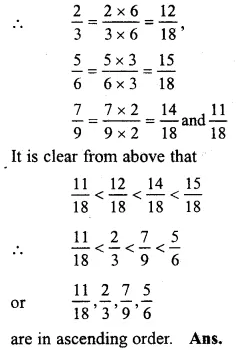Question 20.
Solution:
$$\frac { 2 }{ 5 } ,\frac { 7 }{ 10 } ,\frac { 11 }{ 15 } and\frac { 17 }{ 30 }$$
LCM of 5, 10, 15 and 30 = 30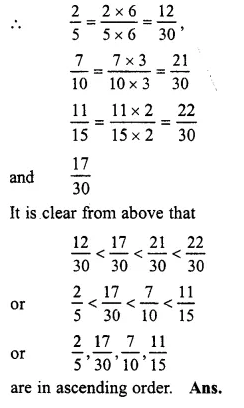Question 21.
Solution:
$$\frac { 3 }{ 4 } ,\frac { 7 }{ 8 } ,\frac { 11 }{ 16 } and\frac { 23 }{ 32 }$$
LCM of 4, 8, 16 and 32 = 32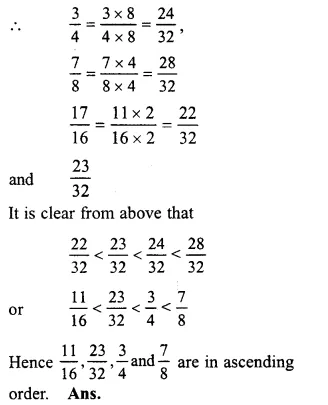Arrange the following fractions in the descending order :

Question 22.
Solution:
$$\frac { 3 }{ 4 } ,\frac { 5 }{ 8 } ,\frac { 11 }{ 12 } and\frac { 17 }{ 24 }$$
LCM of 4, 8, 12 and 24 = 24Question 23.
Solution:
$$\frac { 7 }{ 9 } ,\frac { 5 }{ 12 } ,\frac { 11 }{ 18 } and\frac { 17 }{ 36 }$$
LCM of 9, 12, 18 and 36 = 36Question 24.
Solution:
$$\frac { 2 }{ 3 } ,\frac { 3 }{ 5 } ,\frac { 7 }{ 10 } and\frac { 8 }{ 15 }$$
LCM of 3, 5, 10 and 15 = 30Question 25.
Solution:
$$\frac { 5 }{ 7 } ,\frac { 9 }{ 14 } ,\frac { 17 }{ 21 } and\frac { 31 }{ 42 }$$
LCM of 7, 14, 21 and 42 = 42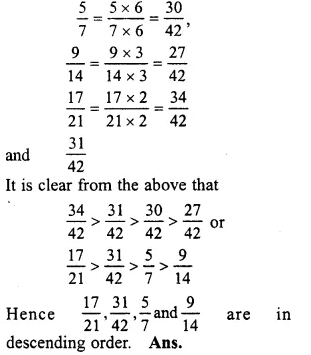Question 26.
Solution:
∴ the numerators are equal
∴ The fraction having small denominator is greater than the fraction having large denominator
∴ In descending order, we can write
$$\frac { 1 }{ 12 } ,\frac { 1 }{ 23 } ,\frac { 1 }{ 7 } ,\frac { 1 }{ 9 } ,\frac { 1 }{ 17 } ,\frac { 1 }{ 50 }$$

Question 27.
Solution:
Here, the numerators of all fractions are equal
∴ The fraction having small denominator is greater than the fraction having large denominator
Now in descending order is
$$\frac { 3 }{ 4 } ,\frac { 3 }{ 5 } ,\frac { 3 }{ 7 } ,\frac { 3 }{ 11 } ,\frac { 3 }{ 13 } ,\frac { 3 }{ 17 }$$

Question 28.
Solution:
Lalita read 30 pages of a book containing 100 pages
She read $$\\ \frac { 30 }{ 100 }$$ = $$\\ \frac { 3 }{ 10 }$$ part of the book and Sarita read $$\\ \frac { 2 }{ 5 }$$ of the book
Now in $$\\ \frac { 3 }{ 10 }$$ and $$\\ \frac { 2 }{ 5 }$$, LCM of 10, 5 = 10
$$\\ \frac { 3 }{ 10 }$$ = $$\\ \frac { 3 }{ 10 }$$
$$\\ \frac { 2 }{ 5 }$$ = $$\\ \frac { 2\times 2 }{ 5\times 2 }$$ = $$\\ \frac { 4 }{ 10 }$$
as $$\\ \frac { 4 }{ 10 }$$ or $$\frac { 2 }{ 5 } >\frac { 3 }{ 10 }$$

Question 29.
Solution:
Rafiq exercised for $$\\ \frac { 2 }{ 3 }$$ hour and Rohit exercised for $$\\ \frac { 3 }{ 4 }$$ hour
In $$\\ \frac { 2 }{ 3 }$$ and $$\\ \frac { 3 }{ 4 }$$, LCM of 3 and 4 = 12
$$\\ \frac { 2 }{ 3 }$$ = $$\\ \frac { 2\times 4 }{ 3\times 4 }$$ = $$\\ \frac { 8 }{ 12 }$$
$$\\ \frac { 3 }{ 4 }$$ = $$\\ \frac { 3\times 3 }{ 4\times 3 }$$ = $$\\ \frac { 9 }{ 12 }$$
$$\frac { 9 }{ 12 } >\frac { 8 }{ 12 }$$
=> $$\frac { 3 }{ 4 } >\frac { 2 }{ 3 }$$
∴ Rohit exercised more time

Question 30.
Solution:
In VI A, 20 student passed out of 25 or $$\\ \frac { 20 }{ 25 }$$ or $$\\ \frac { 4 }{ 5 }$$ students passed
But in VI B, 24 out of 30 passed 24 or $$\\ \frac { 24 }{ 30 }$$ or $$\\ \frac { 4 }{ 5 }$$ students passed
Now $$\\ \frac { 4 }{ 5 }$$ = $$\\ \frac { 4 }{ 5 }$$
∴ Both sections gave same result

Hope given RS Aggarwal Solutions Class 6 Chapter 5 Fractions Ex 5D are helpful to complete your math homework.

If you have any doubts, please comment below. Learn Insta try to provide online math tutoring for you.

## RS Aggarwal Class 6 Solutions Chapter 3 Whole Numbers Ex 3C

These Solutions are part of RS Aggarwal Solutions Class 6. Here we have given RS Aggarwal Solutions Class 6 Chapter 3 Whole Numbers Ex 3C.

Other Exercises

Question 1.
Solution: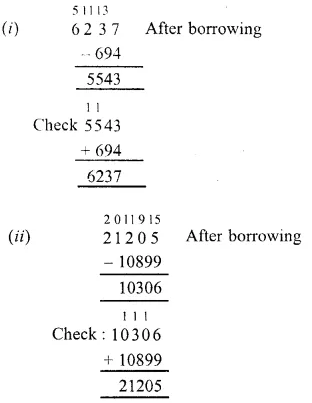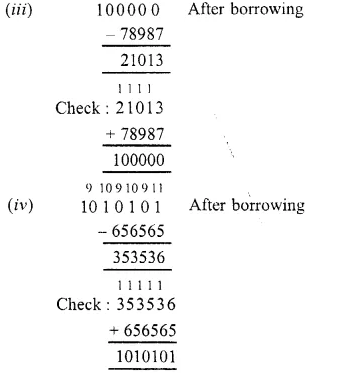Question 2.
Solution: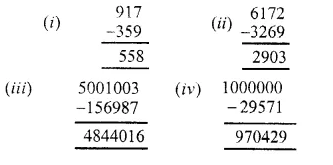Question 3.
Solution:
(i) 463 – 9
= 464 – 1 – 9
= 464 – 10
= 454
(ii) 5632 – 99
= 5632 – 100 + 1
= 5633 – 100
= 5533
(iii) 8640 – 999
= 8640 – 1000 + 1
= 8641 – 1000
= 7641
(iv) 13006 – 9999
= 13006 – 10000 + 1
= 13007 – 10000
= 12007

Question 4.
Solution:
Smallest number of 7-digits = 1000000
Largest number of 4-digits = 9999
Required difference = (1000000 – 9999)
= 990001

Question 5.
Solution:
Deposit in the beginning = Rs. 136000
Next day withdrew = Rs. 73129
Amount left in the bank account = Rs. 136000 – 73129
= Rs. 62871

Question 6.
Solution:
Amount withdrawn from the bank = Rs. 1,00,000
Cost of TV set = Rs. 38750
Cost of refrigerator = Rs. 23890
Cost of jewellery = Rs. 35560
Total amount spent on her purchase = Rs. (38750 + 23890 + 35560)
= Rs. 98200
Amount left with her – Rs. 1,00,000 – Rs. 98200
= Rs. 1800

Question 7.
Solution:
Population of a town = 110500
Increase in 1 year = 3608
Persons left or died = 8973
The population at the end of year = 110500 + 3608 – 8973
= 114108 – 8973
= 105135

Question 8.
Solution:
(i) We have n + 4 = 9
n = 9 – 4 = 5
n = 5
(ii) n + 35 = 101
Subtracting 35 from both sides
n + 35 – 35 = 101 – 35
=> n = 66
n = 66
(iii) n – 18 = 39
n – 18 + 18 = 39 + 18
=> n = 57
n = 57
(iv) n – 20568 = 21403 Adding 20568 to both sides
n – 20568 + 20568 = 21403 + 20568
n = 41971

Hope given RS Aggarwal Solutions Class 6 Chapter 3 Whole Numbers Ex 3C are helpful to complete your math homework.

If you have any doubts, please comment below. Learn Insta try to provide online math tutoring for you.

## RS Aggarwal Class 6 Solutions Chapter 3 Whole Numbers Ex 3B

These Solutions are part of RS Aggarwal Solutions Class 6. Here we have given RS Aggarwal Solutions Class 6 Chapter 3 Whole Numbers Ex 3B.

Other Exercises

Question 1.
Solution:
(i) 458 + 639 = 639 + 458 (Commulative law)
(ii) 864 + 2006 = 2006 + 864 (Commulative law)
(iii) 1946 + 984 = 984 + 1946 (Commulative law)
(iv) 8063 + 0 = 8063 (Additive property of zero)
(v) 53501 + (574 + 799) = 574 + (53501 + 799) (Associative law)

Question 2.
Solution:
(i) 16509 + 114 = 16623
Check : 16623 – 114 = 16509 which is given.
(ii) 2359 + 548 = 2907
Check: 2907 – 2359 = 548 which is given
(iii) 19753 + 2867 = 22620
Check : 22620 – 19753 = 2867 which is given

Question 3.
Solution:
(1546 + 498) + 3589
= 2044 + 3589
= 5633
and 1546 + (498 + 3589)
= 1546 + 4087
= 5633
Yes, the above two sum are equal.
The property used is associative law of addition.

Question 4.
Solution:
(i) 953 + 707 + 647
= (953 + 647) + 707
(by associative law)
= 1600 + 707
= 2307
(ii) 1983 + 647 + 217 + 353
= (1983 + 217) + (647 + 353)
= 2200 + 1000 = 3200
(iii) 15409 + 278 + 691 + 422
= (15409 + 691) + (278 + 422)
(by associative law)
= 16100 + 700
= 16800
(iv) 3259 + 10001 + 2641 + 9999
= (3259 + 2641) + (10001 + 9999)
(by associative law)
= 5900 + 20000
= 25900
(v) 1 +2 + 3 +4 + 96 + 97 + 98 + 99
= (1 +99) + (2 + 98) + (3 + 97) + (4 + 96)
= (100+ 100) + (100 +100)
= 200 + 200
= 400
(vi) 2 + 3 + 4 + 5 + 45 + 46 + 47 + 48
= (2 + 48) + (3 + 47) + (4 + 46) + (5 + 45)
= (50 + 50) + (50 + 50)
= 100 + 100 = 209

Question 5.
Solution:
(i) 6784 + 9999 = (6784 – 1) + (9999 + 1)
= 6783 + 10000
= 16783
(ii) 10578 + 99999
= (10578 – 1) + (99999 + 1)
= 10577 + 100000
= 110577

Question 6.
Solution:
Yes it is true, by the property of associative law of addition.

Question 7.
Solution:
The magic squares given are completed as under:In each row/column, the sum = 46

Question 8.
Solution:
(i) The sum of two odd numbers is an odd number (F)
As sum of two odd numbers is alway an even number
(ii) The sum of two even number is an even number (T)
(iii) The sum of an even number and an odd number is an odd number (T)

Hope given RS Aggarwal Solutions Class 6 Chapter 3 Whole Numbers Ex 3B are helpful to complete your math homework.

If you have any doubts, please comment below. Learn Insta try to provide online math tutoring for you.